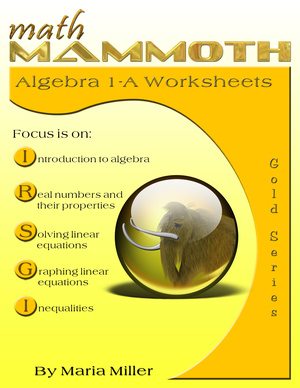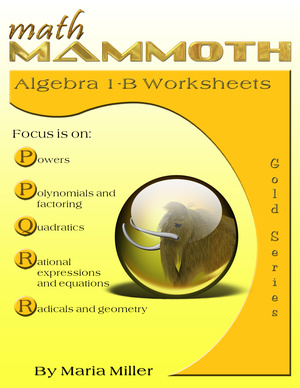^
You are here: HomeGolden Series → Algebra 1

# Math Mammoth Algebra 1 Worksheets82 pages
(74 worksheets)70 pages
(63 worksheets)

Price: \$13.00
(includes both 1-A and 1-B and their answer keys)

Sample pages (PDF)

Note: This is a legacy product and no longer updated.

Math Mammoth Algebra 1 Worksheet Collection consists of two workbooks, 1-A and 1-B, covering all typical topics of algebra 1 course. The worksheets have been created especially for teachers: each problem sheet is one page, concentrating on one topic.

Please note that these are worksheets collections and do not contain textbook explanations. So if you are a student, you cannot use these alone to learn algebra. (See some suggestions for algebra curriculum here.)

These algebra worksheets have been "handicrafted" one by one. Each worksheet is on one topic, but contains varying problems about that topic. The problems include some that lead to a concept, basic practice problems about the topic on hand, and most worksheet also include 1-2 challenging problems.

This collection contains a lot of word problems. In essence, the worksheets are like the problem section of a math book, and far from the mechanical worksheets created by a script.

 1-A covers - Introduction to Algebra - Real Numbers and Their Properties - Solving Linear Equations - Graphing Linear Equations and Functions - Solving and Graphing Linear Inequalities - Systems of Linear Equations and Inequalities 1-B covers - Powers - Polynomials and Factoring - Quadratic Equations (Inc. graphing) - Rational Expressions and Equations - Radicals and Geometry

## Math Mammoth Algebra 1-A

The collection 1-A covers the first half of a typical algebra 1 course topics. The first section of the book contains introductory problems about variables, expressions, translating words into symbols, and building equations.

The second section covers basic properties of real numbers. Next follows a section on solving linear equations, which also covers ratio, proportion, and percent problems. I have also included lots of word problems here.

The next section, Graphing Linear Equations and Functions, starts with problems about relations, then has worksheets for all the graphing-related concepts, such as slope and parallel and perpendicular lines. Lastly in this section I have included three worksheets about modeling with linear equations.

The next section concentrates on solving and graphing linear inequalities including absolute value inequalities. Lastly in this collection we have systems of linear equations and inequalities.

## Math Mammoth Algebra 1-B

This collection covers the latter half of a typical algebra 1 course topics, starting with exponents and powers, and introductory problems on exponential functions. Next follows a section on polynomials and various factoring techniques.

The next section, Quadratic Equations, includes graphing quadratic functions, solving quadratic equations, various applications (word problems), and using the discriminant. Then comes a section called Rational Expressions and Equations, and the last section covers radical expressions, radical equations, Pythagorean theorem and its applications.

This collection also contains 8 review worksheets that cover the entire algebra course.

See also Linear Functions I and Linear Functions II workbooks from the Make It Real Learning series.

## Better Yet - Bundle Deals!

*BONUS*: Buy any bundle listed above, and get the fully upgraded Soft-Pak software for FREE (includes 4 math and 2 language arts programs, complete with on screen and printable options).

## How and where to order

You can buy Math Mammoth books at:

• Here at MathMammoth.com website — simply use the "Add to cart" buttons you see on the product pages.
• Rainbow Resource carries printed copies for the Light Blue series books, plus several CDs (Light Blue and Blue series).
• At Teachers Pay Teachers you can purchase the Light Blue Series downloads, plus topical units.
• Lulu sells printed copies for most of the Math Mammoth materials (various series).

By purchasing any of the books, permission IS granted for the teacher (or parent) to reproduce this material to be used with his/her students in a teaching situation; not for commercial resale. However, you are not permitted to share the material with another teacher.

In other words, you are permitted to make copies for the students/children you are teaching, but not for other teachers' usage.

Math Mammoth books are PDF files. You will need Adobe Reader to view them, including if you use a Mac or Linux. You can try other PDF viewers, but they seem to either omit or mess up some of the images.
WAIT!

Receive my monthly collection of math tips & resources directly in your inbox — and get a FREE Math Mammoth book!You can unsubscribe at any time.

### Math Mammoth Tour

Confused about the different options? Take a virtual email tour around Math Mammoth! You'll receive:

An initial email to download your GIFT of over 400 free worksheets and sample pages from my books. Six other "TOURSTOP" emails that explain the important things and commonly asked questions concerning Math Mammoth curriculum. (Find out the differences between all these different-colored series!)

This way, you'll have time to digest the information over one or two weeks, plus an opportunity to ask me personally about the curriculum.
A monthly collection of math teaching tips & Math Mammoth updates (unsubscribe any time)### "Mini" Math Teaching Course

This is a little "virtual" 2-week course, where you will receive emails on important topics on teaching math, including:

- How to help a student who is behind
- Troubles with word problems
- Teaching multiplication tables
- Why fractions are so difficult
- The value of mistakes
- Should you use timed tests
- And more!

A GIFT of over 400 free worksheets and sample pages from my books right in the very beginning.A monthly collection of math teaching tips & Math Mammoth updates (unsubscribe any time)
Enter your email to receive math teaching tips, resources, Math Mammoth news & sales, humor, and more! I tend to send out these tips about once monthly, near the beginning of the month, but occasionally you may hear from me twice per month (and sometimes less often).• A GIFT of over 400 free worksheets and sample pages from my books.